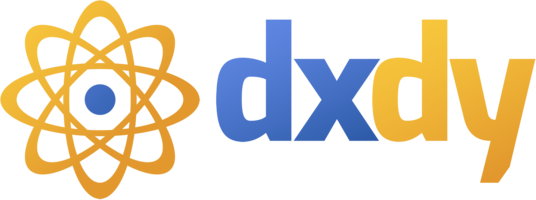# Научный форум dxdy

Математика, Физика, Computer Science, Machine Learning, LaTeX, Механика и Техника, Химия,
Биология и Медицина, Экономика и Финансовая Математика, Гуманитарные науки

 Сравнение альтернатив Matlab (Scilab, Octave, FreeMat)
 Заслуженный участник15/05/05
3433
USA
 Последний раз редактировалось Yuri Gendelman 21.05.2011, 21:06, всего редактировалось 1 раз. Информация к размышлению."A Comparative Evaluation of Matlab, Octave, FreeMat, and Scilab for Research and Teaching"by Neeraj Sharma and Matthias K. GobbertTechnical Report number HPCF-2010-7,University of Maryland, Baltimore County, 2010.Этот 39-страничный технический отчет посвящен сравнительной оценке бесплатных аналогов коммерческого пакета Matlab.Аннотация:Цитата:The commercial software package Matlab is the most widely used numerical computational package. GNU Octave, FreeMat, and Scilab are other numerical computational packages that have many of the same features as Matlab. Unlike Matlab, the other three packages are free of charge to the user and easily available to download to Linux, Windows, or Mac OS X operating systems. Are these packages viable alternatives to Matlab for uses in research or teaching? We review in depth the basic operations of Matlab, such as solving a system of linear equations, computing eigenvalues and eigenvectors, and plotting in two-dimensions. In addition, we research a more complex test problem resulting from a finite difference discretization of the Poisson equation in two spatial dimensions. Then, we compare the results we receive from GNU Octave, FreeMat, and Scilab to our previously found Matlab results. All packages gave identical numerical results, though Scilab exhibited a limitation in the size of the linear system it could solve in the complex test problem and FreeMat was hampered by the lack of a conjugate gradient function. The available graphical functions differ in functionality, but give equivalent plots, though FreeMat has limited three-dimensional graphics capabilities at present. Based on these tests, we conclude that GNU Octave is the most compatible with Matlab due to its numerical abilities and the similarity of its syntax. Another reason to consider Octave is that free parallel computing extensions are available that are known to work with this package. Therefore, for uses in research, Octave’s maturity and resulting richness of functions make it a viable alternative to Matlab. For uses in teaching, the similarity of its syntax with Matlab will likely make textbook instructions directly applicable, and we thus recommend consideration of Octave for this purpose, though the particular assignments of each course will need to be tested in detail.Re: Сравнение альтернатив Matlab (Scilab, Octave, FreeMat)
 Заслуженный участник11/04/08
2552
Физтех
 Yuri GendelmanСпасибо! Надо посмотреть на этот GNU Octave, раз он такой крутой.Показать сообщения за: Все сообщения1 день7 дней2 недели1 месяц3 месяца6 месяцев1 год Поле сортировки АвторВремя размещенияЗаголовок по возрастаниюпо убыванию

Модераторы: Toucan, maxal, PAV, Karan, Супермодераторы

#### Кто сейчас на конференции

Сейчас этот форум просматривают: нет зарегистрированных пользователей

 Вы не можете начинать темыВы не можете отвечать на сообщенияВы не можете редактировать свои сообщенияВы не можете удалять свои сообщенияВы не можете добавлять вложения

 Найти: